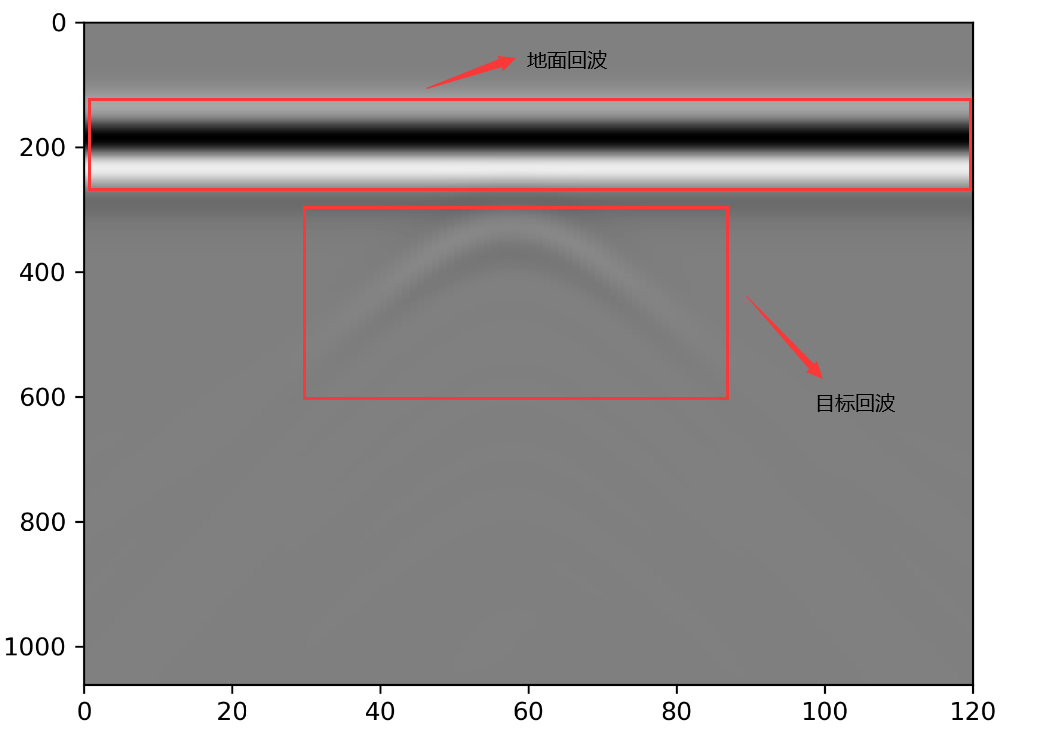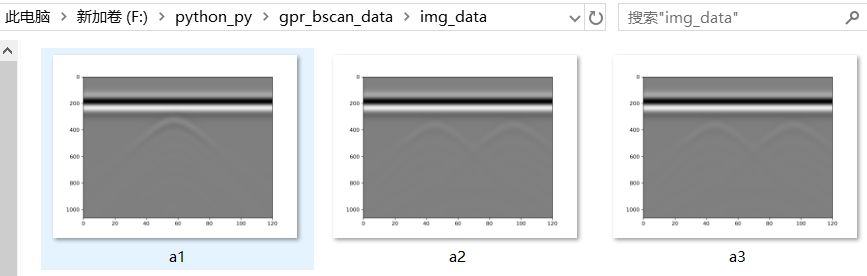# 2.Python脚本

import sys
sys.path.append('D:/my_gprmax/gprMax') #把gprMax安装路径添加至系统，使import可以找到gprMax模块
import os
from gprMax.gprMax import api
import numpy as np
from tools.plot_Bscan import get_output_data, mpl_plot
from tools.outputfiles_merge import merge_files
import matplotlib.pyplot as plt

num_scan = 120 #正演仿真次数（A扫描次数）->B扫描
geo_only = False #是否只生成模型图

path = os.getcwd()#获得当前脚本所在的文件路径
#print(path)
root = path+'/in_data'   #指定输入文件的路径
files = os.listdir(root)   #得到路径下的文件夹名或者文件名，形成列表

for file in files:
if file.endswith('.txt'):  #找到.txt文件
# print(file)
filename = root+'/'+file  #得到文件名的绝对路径
fi = filename[0:-4]        #去掉文件名后的.txt后缀,注意保留了前面的路径
api(filename, n=num_scan, geometry_only=geo_only) #geometry_only：仅几何图形
merge_files(fi, removefiles=True)

"""B扫描绘图"""
filename_b = fi+'_merged.out'
rxnumber = 1
rxcomponent = 'Ez'
#获取回波数据
outputdata, dt = get_output_data(filename_b, rxnumber, rxcomponent)
#保存回波数据
fi2 = fi.split('/')[-1]  #得到文件名，去掉了前面的路径
out_path = path+'/out_data'
if (os.path.exists(out_path)):
pass
else:
os.mkdir(out_path)
np.savetxt(out_path+'/'+fi2+'.txt',outputdata,delimiter=' ')#未去除直达波

#绘图
plt.imshow(outputdata, extent=[0, outputdata.shape, outputdata.shape, 0], interpolation='nearest', aspect='auto', cmap='gray',
vmin=-np.amax(np.abs(outputdata)), vmax=np.amax(np.abs(outputdata)))
img_path = path+'/img_data'
if (os.path.exists(img_path)):
pass
else:
os.mkdir(img_path)
plt.savefig(img_path+'/'+fi2+'.png',dpi=300)#保存图片
# plt.show()


# 3.可能出现的报错# 4.数据展示09-30
05-311万+09-11
03-06
05-131万+
09-053211
07-24211
12-3038
12-202666
04-222995
04-22296